﻿ On Proper Classification and Placement of Students in Nigerian University Systems Using Discriminant Analysis

### On Proper Classification and Placement of Students in Nigerian University Systems Using Discriminant...

G. O. Nwafor, C. E OnwukweOPEN ACCESSPEER-REVIEWED

## On Proper Classification and Placement of Students in Nigerian University Systems Using Discriminant Analysis

G. O. Nwafor1,, C. E Onwukwe2

1Department of Mathematics and Statistics, Cross River University of Technology Calabar, Nigeria

2Department of Mathematics, Statistics and Computer Science, University of Calabar, Cross River State, Nigeria

### Abstract

This paper attempts to provide more realistic and reliable way of placing Nigerian students seeking admission into Nigerian University system using Discriminant Analysis and also providing quantitative analysis of a Discriminant Analysis approach to prediction of student’s admission scores into a university system. The paper utilizes secondary data sourced from the Admission and Public Relation Department of Cross Rivers University of Technology Calabar, the conditions for predictive and classification discriminant analysis were obtained, and the empirical result yield a discriminant linear classification function results on the various faculties of interest on the method of admission system obtainable in Nigeria university system. The study reveals university mandatory examination (UME) and Aptitude Test score of students in their various faculties. The linear function established a hit ratio of 83% of which successfully predicted the student admission scores. The study had apparent error rate of 17% which explains the probabilities of misclassification.

• Nwafor, G. O., and C. E Onwukwe. "On Proper Classification and Placement of Students in Nigerian University Systems Using Discriminant Analysis." American Journal of Applied Mathematics and Statistics 2.6 (2014): 394-397.
• Nwafor, G. O. , & Onwukwe, C. E. (2014). On Proper Classification and Placement of Students in Nigerian University Systems Using Discriminant Analysis. American Journal of Applied Mathematics and Statistics, 2(6), 394-397.
• Nwafor, G. O., and C. E Onwukwe. "On Proper Classification and Placement of Students in Nigerian University Systems Using Discriminant Analysis." American Journal of Applied Mathematics and Statistics 2, no. 6 (2014): 394-397.

 Import into BibTeX Import into EndNote Import into RefMan Import into RefWorks

### 1. Introduction

Discriminant Analysis is used in situation where the clusters are known in advance. The aim of discriminate analysis is to classify an observation, or several observations, into these known groups. The admission system adopted in major university based on the education intervention of the kind in higher education has been of great and area for many researcher or educations, over the years.  carried out a study on classification of students into various Departments on the basis of their cumulative results for one year foundation programme otherwise known as pre-national diploma (PRE-ND) in polytechnics system.  carried out a study in application of discriminant analysis to predict the classes of degree for graduating students in a university system. The analyses yield a linear discriminant function which successfully classified or predicted the graduating student’s class of degrees. Discriminant analysis builds a predictive model for group membership the model is composed of a discriminant function (or, for more than two groups, a set of linear classification function) based on linear combination of the predictive variables that proved the best discriminant among groups.  carried out a study to determine of a differentiation or separation among students graduating, withdrawing or failing could be identified.  applied the t-statistic  to investigate how predictable the final years result would be using the first year result or grade point average (GPA) of some selected university graduates. In the past 35 years, research in academic prediction has centered on graduation, withdrawal, failure and selection of the students on the basis of either their collegiate success or cumulative results of remedial or pre- nd, and literature to date suggests no loss of interest.

In the study, our major task is to empirically yield a discriminant linear classification function on the various faculties of interest on the method of admission system obtainable in Nigeria University, the UME and aptitude test score. The first four groups are students from the faculty of science, education, engineering and environmental science with their UME and Aptitude test scores, respectively. This prediction of student’s admission scores into a university system, formed by the discriminant analysis seems more appropriate than commonly used educational measure such as regression, weights etc. Research has show that predictive discriminant analysis perform quite well categorical date  also violations of the assumption underlining regression modeling can have serious repercussions .

### 2. Materials and Methodology

The data type and sources of this study was secondary data from the Admission and Public Relation unit of Cross River University of Technology Calabar. Other augmenting sources of this study include published articles and journals, working papers, textbooks and relevant internet resources. In the first state of data collection, this system of scores was established, the UME SCORE AND APPTITUDE TEST SCORES from the admission unit which includes various department and their respective faculties, but the interest of the researcher was on the faculty level comprising the entire departments. A simple random sampling technique was employed to obtain the desired faculties of four (4) the faculty of science, Engineering, Education and Environmental science. A systematic sampling technique was quantitatively applied to obtain the desired sample size of 150 per faculty in the two systems of admission scores.

2.1. Data Analysis

The method is to set up a procedure based on the students/applicants admission score of the two systems in their respective faculties by the discriminate analysis function and placement to the program by score by the classification function for the groups /faculties. The fisher linear discriminant function  will be adopted in the study since it will discriminate between the groups better than any other linear function .

The analysis yields variance-covariance matrices for the groups are given as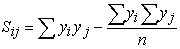(1)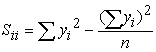(2)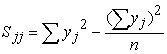(3)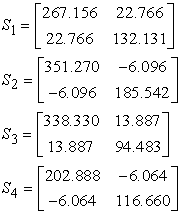And the pooled covariance matrices given as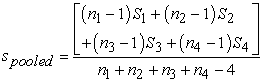(4)The Table 1 indicates the descriptive properties of the study groups.

#### Table 1. Descriptive Statistics

2.1.1. TEST OF SIGNIFICANT

The hypothesis and test statistics is: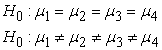With the F-transformation of Hotelling’sgiven(5)

Were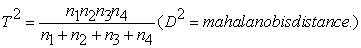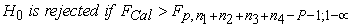This indicates that at 5% level of significant; reject the hypothesis of equality of group means. This implies that there exist significant differences among the group means.

2.1.2. DISCRIMINANT LINEAR CLASSIFICATION FUNCTION

Obtain a linear classification function, which is denoted by(6)

Is maximum (largest).Assigning y to a group using the linear classification function and prior probabilities i.e., the distance function whichis maximum with this rule, the probability of misclassification is minimized and the rule becomes(7)

 Certain facts exist; if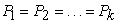then equation (2) which optimizes the classification rate for normal distribution reduces to equation (1) which was based on the heuristic approach of minimizing the distance of y to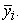APER –The apparent error rate is easily obtained and routinely provided by most classification software programs. It is an estimate of the probability that our classification functions based on the sample will misclassify a future observation.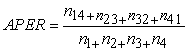(8)

Similarly,

Apparent correction classification rate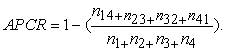(9)

The error and correction classification rate judge the ability of classification procedures to predict group member. The usually use the probability of misclassification which is known as the Aper and its complement Apcr.

### 3. Result

The predictive analytical software (PASW) was employed on the data which yields the following findings and results.

#### Table 2. Linear Classification Function Coefficients

This is the distribution of observation into the gender groups used as a starting point in the analysis. The default prior distribution is the allocation into groups, as seen in the analysis. SPSS allows users to specify different prior with the priors subcommand. The discriminant analysis and classification builds a predictive model for group membership and classification of linear function .The linear classification function coefficients provide the following model for placement of students in their respective programs.Based on the linear combination of the prediction variables that provide the best classification /placement among group of students. The functions are generated from a sample of cases for which group membership is known, the functions can then be applied to new cases that have measurements for the predictor variable but have unknown group membership. This study provides a classification model for placement of student in Cross River University of Technology Calabar.

#### Table 3. Confusion matrix for actual and predicted groups

The study on the discriminant analysis to predict student scores was achieved. The apparent correction rates i.e. the hit ratio yield 83% for the study and probability of misclassification (17%). The Table 3 the confusion matrices which is the measure of predictive ability indicates that discriminant analysis can be used to predict students scores. The linear classification function for the faculties was used to obtain the students aggregate scores for their respective faculties. This quantitative measure of discriminant analysis should be adopted by the study groups to obtain the actual scores for the respective candidates, and having standard cutoff scores by the faculty/university management. The study also reveals the descriptive statistics for the groups of the sampled data. The Table 2 shows the linear discriminant classification function for the study.

### 4. Discussion

The concern for the predictive ability of the linear discriminant function has obscured and even confused the fact that two sets of techniques based on the purposed of analysis exist , i.e., predictive discriminant analysis (PDA) and descriptive discriminant analysis (DDA).The distinction between PDA and DDA is that predictive discriminant analysis focus on classifying subjects into one of several groups (or to predicate group membership), whereas in descriptive discriminant analysis focus on revealing major differences among the groups.  aptly stated the purposes of the two analyses are different; the techniques in the two analyses are different. There is, perhaps, some feasibility of the “mixing of DDA for purpose of corroboration of results. Generally research questions are of the descriptive type or of the predictive type; only seldom would both types of questions be addressed in a given research situation. The two types of discriminant analyses i.e., PDA and DDA have different histories of development. According to  “discriminant analysis for the first three or four decades focused on the prediction of group membership”, PDA, whereas DDA usage did not appear until the 1960s and “its use has been very limited to apply research settings over the past two decades”. Hence, PDA is appropriate when the researcher is interested in assigning units (individuals) to groups based on composites scores on several predictor variables. The accuracy of such prediction can be assessed by examining “hit rates” as against chance; for example, the most basic question answered by PDA is “given the individual scores on several predictor variables, which group represents their true membership group?” Again, the focus of PDA is predication and the accuracy of hit rates. As  noted with respect to PDA, “one is basically interested in determining a classification rule and assessing its accuracy”.

### References

  Usoro A. E “Discriminant analysis and its application to the classification students on the basis of their academic performances” J. Res. Phy. Sci. 2(3):53-55. 2006.In article  Erimafa J.T, Iduseri A. and Edokpa I. W “Application of discriminant analysis to predict the class of degree for graduating students in a university” International journal of physical science vol.4 (1), pp.016-021. 2009.In article  Charles B K and June E H “predicting graduation withdrawal and failure in college by multiple discriminant analysis” J. Edu. Measure 7(2):91-95.1970.In article  Adebayo SB and Jolayemi ET “Effect of rare outcome on the measure of agreement index” j. Nig. Stat. assoc. 13:1:10. 1990.In article  Adebayo SB and Jolayemi E T “on the effect of rare outcome on some agreements/Concordance indices” Nig. J pure and appl. Sci. 13:718-723. 1998.In article  Gilbert E S “on discriminant using qualitative variable” J. Amr Stat Soc. Pp 399-4d4. 1968.In article  Cook RD and Weisbery S “Residuals and influence in regression:” London; chapman and hall.1982.In article  Fisher R A “the use of multiple measurements in taxonomic problems Ann. Eug” 7:179-188. 1936.In article CrossRef  Anderson T W “an introduction to multivariate statistical analysis” New York john wiley. 1958.In article  Alvin C. Rencher “Methods of multivariate Analysis.” John Wiley & Son. Inc. Publication. 2002.In article  Huberty C J, Barton R M “an introduction to discriminant analysis Measurement and evaluations in counseling and development” 22: 158-168. 1989.In article  Hubert C J “Applied discriminant analysis” New York Wiley and sons. 1994.In article